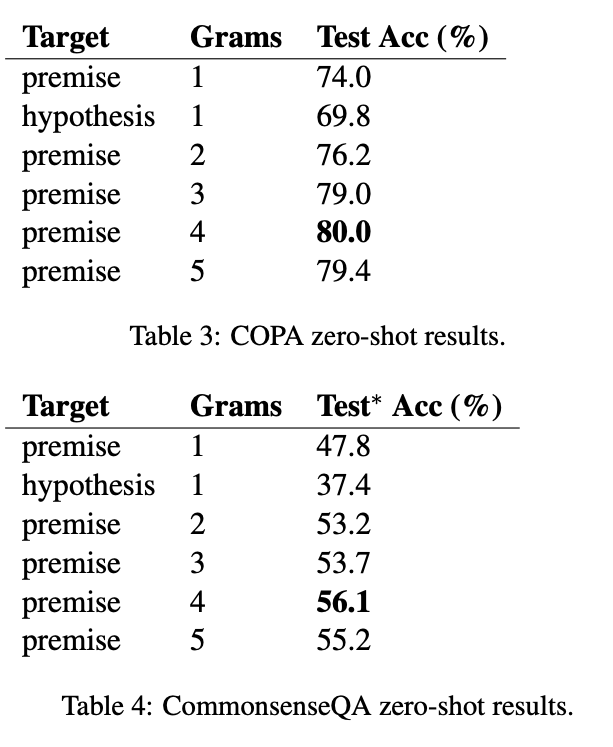# Pre-training Is (Almost) All You Need: An Application to Commonsense Reasoning

Pre-trained transformers might have already learned an intrinsic textual representation of a task without fine-tuning on that task. The authors proposed a learning-to-rank loss function tailored for plausibility ranking and achieved comparable results to supervised approaches.

## Plausibility Ranking Task

Match a set of hypotheses to a given premise.

Given an input premise p=(p^{(1)},p^{(2)},\dots,p^{(L_{p})}), and a set of candidate hypotheses:
H=\left\lbrace h_{i} = (h_{i}^{(1)},h_{i}^{(2)}, \dots, h_{i}^{(L_{i})}) \right\rbrace_{i=1 \dots n}, we aim to identify the fitting hypothesis h^{*} \in H which correctly matches p.

One common baseline is binary classification of each (p, h) pair. However, the authors show that their scoring function works much better when dataset is small.

## Approach

The basic idea is to use the log likelihood of masked premise and hypothese as their score, then take the one with maximum score as the prediction.

### Encoder

Note that [SEP] is not used between p and h, which is referred to as full-text format in this paper.

### Scoring Function

S^{p}_{i} = \sum_{k=1}^{L_{p}} \log \left[ P \left( p^{(k)} \mid \mathbf{s}_{i}^{\backslash p^{(k)}}\right)\right],

### Decision Function

\begin{align} h^{*} = h_{j} \in H \;\; s.t. \;\; \max_{i=1\dots n} S^{p}_{i} = S^{p}_{j}. \end{align}

### Loss Function

In supervised setting, the author proposed a margin-based loss:

\mathcal{L} = \frac{1}{n} \sum_{\substack{i=1 \\ i\neq i^{*}}}^{n} \max\left( 0, \eta - S^{p}_{i^{*}} + S^{p}_{i} \right),

## Results

### Unsupervised (zero-shot)## Comments

I would personally rate this paper as:

• 5: Transformative: This paper is likely to change our field. It should be considered for a best paper award.
• 4.5: Exciting: It changed my thinking on this topic. I would fight for it to be accepted.
• 4: Strong: I learned a lot from it. I would like to see it accepted.
• 3.5: Leaning positive: It can be accepted more or less in its current form. However, the work it describes is not particularly exciting and/or inspiring, so it will not be a big loss if people don’t see it in this conference.
• 3: Ambivalent: It has merits (e.g., it reports state-of-the-art results, the idea is nice), but there are key weaknesses (e.g., I didn’t learn much from it, evaluation is not convincing, it describes incremental work). I believe it can significantly benefit from another round of revision, but I won’t object to accepting it if my co-reviewers are willing to champion it.
• 2.5: Leaning negative: I am leaning towards rejection, but I can be persuaded if my co-reviewers think otherwise.
• 2: Mediocre: I would rather not see it in the conference.
• 1.5: Weak: I am pretty confident that it should be rejected.
• 1: Poor: I would fight to have it rejected.

### zero-shot

I know pretrained MLM encodes lots of knowledge but don’t know to which extent it encodes. This paper shows that on some dataset it can be very close to fine-tuned models.

### MLM Likelihood

Their scoring function is quite novel to me and possibly can be used in other language modeling scenarios too.